A point-mass lens and a point-mass source

In the specific case of a point-mass lens and a point-mass source the deflection angle is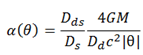and the deflection potential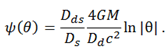With these we get that the lens equation is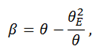where we have used the Einstein’s angleIn this case there are always two point-images,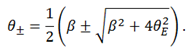Using the deflection potential we get that there is only a critical curve, a ring with θ=θE. The corresponding caustic is point-like, located in β=0. The magnification of each image is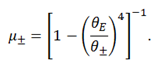Although the magnification is the brightness of each image, in this case it appears as the size of the image. That is done to emphasize the fact that when the source is very far from the lens (in the plane of the sky) the observer only sees one image with the same shape and size of the source, because the other has zero brightness. When the source is on the caustic, the images are infinitely magnified, so in this case we see that they get really big.

We have taken G/c2=1 and 1 light-year=1. As the angles are really small we multiply them by 107, which simulates the effect of looking through a telescope.